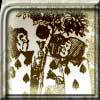#### You may also like### Card Trick 2

Can you explain how this card trick works?### Painting Cubes

Imagine you have six different colours of paint. You paint a cube using a different colour for each of the six faces. How many different cubes can be painted using the same set of six colours?### Cube Paths

Given a 2 by 2 by 2 skeletal cube with one route `down' the cube. How many routes are there from A to B?

# Cinema Costs

##### Age 11 to 14 ShortChallenge Level

Finding combinations which add up to whole numbers of pounds
$2\times70\text p+ 3\times20\text p$ is a whole number of pounds
$2$ adults $+$ $3$ children $= £15.40+ £12.60$
$= £28$
But $£28\times3$ is less than $90$ and $28\times4$ is more than $100$

$10$ adults $= £77$
$5$ children $= £21$

$77+21=98$ too big
$21\times3 + 28 = 91$
$(3$ lots of $5$ children$) + (2$ adults $+$ $3$ children$) = £91$

Using multiples of $70\text{p}$
Both $£4.20$ and $£7.70$ are multiples of $70p$, so $£C$ must also be a multiple of $70p$. The only multiple of $70p$ between $£90$ and $£96$ which is a whole number of pounds is $£91$.

$7$ children and $8$ adults cost:
$7\times £4.20+8\times £7.70= £29.40+ £61.60= £91$.

Or

$18$ children and $2$ adults cost:
$18\times £4.20 + 2\times £7.70 = £75.60 + £15.40 = £91$.

This problem is taken from the UKMT Mathematical Challenges.
You can find more short problems, arranged by curriculum topic, in our short problems collection.